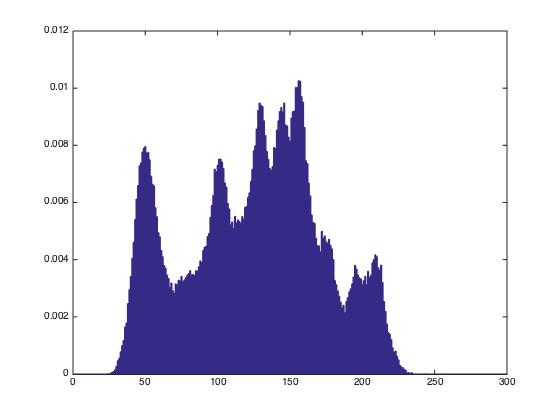# 图像编码与压缩

## Huffman编码

Posted by     Keyon                      on November 29, 2018

1. 统计该图像的概率直方图，并画出直方图
2. 计算该图像的熵
3. 对其进行霍夫曼编码
4. 分别计算压缩率和冗余度

``````clc,clf,clear all,close all;

imshow(I,[]);  % 显示图像
L = 256;

%获取各符号的概率
[M,N] = size(I);
I0 = I(:);
P = zeros(1,L);
for i = 0:L-1
P(i+1) = length(find(I0 == i))/(M*N);
end

%统计概率直方图
figure;bar(P);

% 计算该图像的熵
H = entropy(I);

% 进行霍夫曼编码
code = huffman(P);

% 计算压缩率和冗余度
Lc = 0;
for i = 1:L
Lc = Lc+P(i)*length(code{i,1});%平均码长
end
m = log2(L);
C = m/Lc;  %压缩比
R = 1-H/Lc; %冗余度
R = strcat(num2str(R*100),'%');%冗余度的百分比表示
``````

``````function h = entropy(x, n)
%ENTROPY Computes a first-order estimate of the entropy of a matrix.
%   H = ENTROPY(X, N) returns the first-order estimate of matrix X
%   with N symbols (N = 256 if omitted) in bits/symbol. The estimate
%   assumes a statistically independent source characterized by the
%   relative frequency of occurrence of the elements in X.

%   Copyright 2002-2004 R. C. Gonzalez, R. E. Woods, & S. L. Eddins
%   Digital Image Processing Using MATLAB, Prentice-Hall, 2004
%   \$Revision: 1.4 \$  \$Date: 2003/10/26 18:35:35 \$

error(nargchk(1, 2, nargin));         % Check input arguments
if nargin < 2
n = 256;                           % Default for n.
end

x = double(x);                        % Make input double
xh = hist(x(:), n);                   % Compute N-bin histogram
xh = xh / sum(xh(:));                 % Compute probabilities

% Make mask to eliminate 0's since log2(0) = -inf.
i = find(xh);

h = -sum(xh(i) .* log2(xh(i)));       % Compute entropy
``````

``````function CODE = huffman(p)
% HUFFMAN Builds a variable-length Huffman code for a symbol source.
%   CODE = HUFFMAN(P) returns a Huffman code as binary strings in
%   cell array CODE for input symbol probability vector P. Each word
%   in CODE corresponds to a symbol whose probability is at the
%   corresponding index of P.
%
%   Based on huffman5 by Sean Danaher, University of Northumbria,
%   Newcastle UK. Available at the MATLAB Central File Exchange:
%   Category General DSP in Signal Processing and Communications.

%   Copyright 2002-2004 R. C. Gonzalez, R. E. Woods, & S. L. Eddins
%   Digital Image Processing Using MATLAB, Prentice-Hall, 2004
%   \$Revision: 1.5 \$  \$Date: 2003/10/26 18:37:16 \$

% Check the input arguments for reasonableness.
error(nargchk(1, 1, nargin));
if (ndims(p) ~= 2) | (min(size(p)) > 1) | ~isreal(p) | ~isnumeric(p)
error('P must be a real numeric vector.');
end

% Global variable surviving all recursions of function 'makecode'
global CODE
CODE = cell(length(p), 1);  % Init the global cell array

if length(p) > 1            % When more than one symbol ...
p = p / sum(p);          % Normalize the input probabilities
s = reduce(p);           % Do Huffman source symbol reductions
makecode(s, []);         % Recursively generate the code
else
CODE = {'1'};            % Else, trivial one symbol case!
end;

%-------------------------------------------------------------------%
function s = reduce(p);
% Create a Huffman source reduction tree in a MATLAB cell structure
% by performing source symbol reductions until there are only two
% reduced symbols remaining

s = cell(length(p), 1);

% Generate a starting tree with symbol nodes 1, 2, 3, ... to
% reference the symbol probabilities.
for i = 1:length(p)
s{i} = i;
end

while numel(s) > 2
[p, i] = sort(p);    % Sort the symbol probabilities
p(2) = p(1) + p(2);  % Merge the 2 lowest probabilities
p(1) = [];           % and prune the lowest one

s = s(i);            % Reorder tree for new probabilities
s{2} = {s{1}, s{2}}; % and merge & prune its nodes
s(1) = [];           % to match the probabilities
end

%-------------------------------------------------------------------%
function makecode(sc, codeword)
% Scan the nodes of a Huffman source reduction tree recursively to
% generate the indicated variable length code words.

% Global variable surviving all recursive calls
global CODE

if isa(sc, 'cell')                   % For cell array nodes,
makecode(sc{1}, [codeword 0]);    % add a 0 if the 1st element
makecode(sc{2}, [codeword 1]);    % or a 1 if the 2nd
else                                 % For leaf (numeric) nodes,
CODE{sc} = char('0' + codeword);  % create a char code string
end
``````• 熵：H = 7.4471
• 压缩比：C = 1.0709
• 冗余度：R = 0.31214%